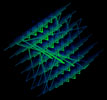cubeLife.org

# Magic matrices: a definition

Magic squares are symmetrical matrices consisting of regular sequences of integers, arranged in two dimensions so that the total of all the numbers in every line produces an identical sum. Typically, the numbers run from 1-n where n represents the 'order' of the square or cube. For instance, in a magic cube of order 3 there will be 3 x 3, or 9, numbers running from 1 - 9.

Magic cubes are similar matrices expanded into three dimensions, while Magic hyper-cubes extend the principle of the magic cube into more than three dimensions. Magic hyper-cubes share many properties with magic squares and cubes.

There are other kinds of 'magic' arrangements of number, such as anti-magic squares, magic stars, matrices of various meaningful non-contiguous integer sequences (such as consecutive primes) and other arrangements. Collectively, this kind of object can be called a magic matrix.

The n x n matrix of a magic square (or n x n x n matrix of a magic cube... etc.) of n numbers per line can be arranged in a finite number of ways.

Disregarding the 1 x 1 square (there is no 2 x 2 magic square), the smallest magic square - the 3 x 3 - has only one solution. This solution may be reflected and rotated in 8 ways while maintaining the relationship of the numbers to each other; however, most solutions exclude these reflections and rotations, which are seen as trivial to the solution of the problem.

There are 880 permutations of the order 4 magic square, and properties within subsets of the order 4 'family' are still being defined.

The number of possible solutions increases rapidly with each number until, from order 6 upwards the (huge) number of permutations can currently only be estimated.